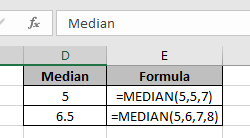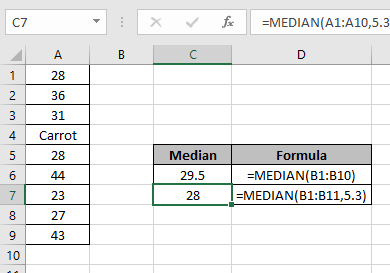# How to use the Excel MEDIAN function

The MEDIAN function is a built-in Excel mathematical function. Median of a range of numbers is the middle value or middle number.
Syntax:

=MEDIAN(number1, [number2],...)

Note: Text, Logic_test and empty cells are ignored by the function.

Median of values : 5,5,7 : 5 (middle number)
Median of values : 5,6,7,8 : 6.5 (middle value)Now use the excel Median function on the range of valuesIn the C7 cell, we just added one more number (5.3) in the range of numbers (A1:A10).

Here we got the median of the range of values(A1:A10).

As you can see from the above examples that how to use median function in Excel.

Hope you understood how to use the MEDIAN function in Excel. Explore more articles on Excel Mathematical function here. Please state your queries in the comment box below.

Related Articles:

Excel MODE function

Excel MEAN function

Create Histograms in Excel 2016/2013/2010

Regressions in excel

Popular Articles:

50 Excel Shortcuts to Increase Your Productivity

How to use the VLOOKUP Function in Excel

How to use the COUNTIF function in Excel 2016

How to Use SUMIF Function in Excel

Terms and Conditions of use

The applications/code on this site are distributed as is and without warranties or liability. In no event shall the owner of the copyrights, or the authors of the applications/code be liable for any loss of profit, any problems or any damage resulting from the use or evaluation of the applications/code.